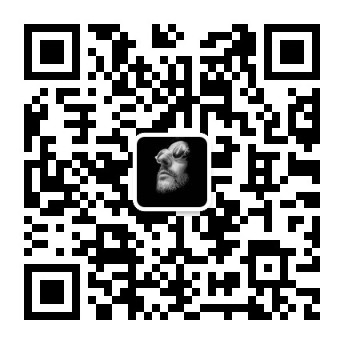# Dart：2.通过一个简单程序来理解Dart基础语法

## 一 . 一个简单的 Dart 程序

// 这是程序执行的入口。
main() {
var number = 42; // 定义并初始化一个变量。
printNumber(number); // 调用一个方法。
}

// 定义个方法。
printNumber(num aNumber) {
print('${str1}${str2}  ${age.toString()}${age} ${age * age}');  输出结果 dart1 darg2 21 dart1 darg2 21 21 441  ### 2 . int 和 double 数字 整形和浮点型  int num1 = 123; double price = 123.452323232; print(price * num1); price = 12; print(price * num1);  输出结果 15184.635757536 1476.0  ### 3 . bool 类型和 if 判断 if 判断只能是 bool 类型的返回值，这点和 js 这衶弱类型语言完全不同：  bool flag = true; if(flag) { print('--- true'); } int num1 = 1; double num2 = 1.0; String num3 = '1'; if(num1 == num2) { print('num1 == num2'); } else { print('num1 != num2'); } if(num1 == num3) { print('num1 == num3'); } else { print('num1 != num3'); } // int a = 1; // if(a) { // 报错 // print('true'); // }  如果 if 使用了非 bool 类型判断报错如下  int a = 1; if(a) { // 报错 print('true'); }  输出结果 [外链图片转存失败,源站可能有防盗链机制,建议将图片保存下来直接上传(img-2bjEzoLy-1571992016907)(E:\Baidu\学习笔记\dart\2.Dart基础介绍\3.png)] ### 4 . 类型判断 is 操作符能够判断类型归属，比如 A is B，能够返回 bool 类型，判断 A 是否属于 B 类型。  var value = 123; if(value is String) { print('${value} is string');
} else if (value is int) {
print('${value} is int'); } else if (value is double) { print('${value} is double');
} else {
print('\${value} is other type');
}


123 is int


### 5 . List（列表）

List 类型是使用非常多的类型，与 js 的 Array 类似，初始赋值可以直接给一个列表，也可以通过 new List() 指定空的列表.

List 作为对象提供了一些的方法和属性： API 文档地址： https://api.dart.dev/dev/2.4.0-dev.0.0/dart-core/List-class.html

List l1 = [123, '123', 'postbird'];
print(l1);
List l2 = new List();
print(l2);
List l3 = new List<String>();
print(l3);
List l4 = new List<int>();
print(l4);
List l5 = new List<int>();
print(l5);
l5.sort();
print(l5);


[123, 123, postbird]
[abc, 123, iterable, 222, 333, 123]
[abc]

[1, 3, 2, 4, 6, 2]
[1, 2, 2, 3, 4, 6]


### 6 . Map 类型

var person = {
'name': 'ptbird',
'age': 24,
'work': ['it1', 'it2']
};
print(person);
print(person.toString());
print(person['name']);
print(person['age']);
print(person['work']);
Map person2 = new Map();
person2['name'] = 'name2';
person2['age'] = 24;
person2['work'] = ['it1', 'it2'];
print(person2);
print(person2['work']);


{name: ptbird, age: 24, work: [it1, it2]}
{name: ptbird, age: 24, work: [it1, it2]}
ptbird
24
[it1, it2]
{name: name2, age: 24, work: [it1, it2]}
[it1, it2]


## 四 . Dart关键字

abstract continue false new this
as default final null throw
assert deferred finally operator true
async do for part try
async dynamic get rethrow typedef
await else if return var
break enum implements set void
case export import static while
catch external in super with
class extends is switch yield
const factory library sync yield

## 五 . 参考资料：

http://dart.goodev.org/guides/language/language-tour